## 2.5.2 Phonon Dispersion Relations of SW-CNTs

The phonon dispersion relations for a SW-CNT can be determined by folding that of a graphene layer (see Section 2.4.2). Since there are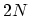carbon atoms in the unit cell of a CNT,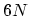phonon dispersion branches for the three-dimensional vibrations of atoms are achieved. The corresponding one-dimensional phonon energy dispersion relation for the CNT is given by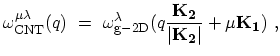(2.12)

where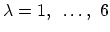denotes the polarization,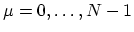is the azimuthal quantum number, and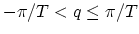is the wave-vector of phonons. However, the zone-folding method does not always give the correct dispersion relation for a CNT, especially in the low frequency region. For example, the out-of-plane tangential acoustic (ZA) modes of a graphene sheet do not give zero energy at the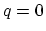when rolled into a CNT. Here, at, all the carbon atoms of the CNT move radially in and out-of-plane radial acoustic vibration, which corresponds to a breathing mode (RBM) with a non-zero frequency . To avoid these difficulties, one can directly diagonalize the dynamical matrix (see Fig. 2.11-a).

Fundamental phonon polarizations in CNTs are radial (R), transverse (T), and longitudinal (L). As shown in Fig. 2.11-b, zone center phonons, also referred to as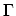-point phonons, can belong to the transverse acoustic (TA), the longitudinal acoustic (LA), the radial breathing mode (RBM), the out-of-plane optical branch (RO), the transverse optical (TO), or the longitudinal optical (LO) phonon branch. The LO phonon branch near the-point has an energy of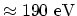, whereas the energy of the RBM phonon branch is inversely proportional to the CNT diameter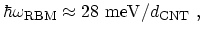(2.13)

where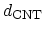is the diameter of the CNT in nanometer [41,42]. Zone boundary phonons, also referred to as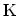-point phonons, are found to be a a mixture of fundamental polarizations .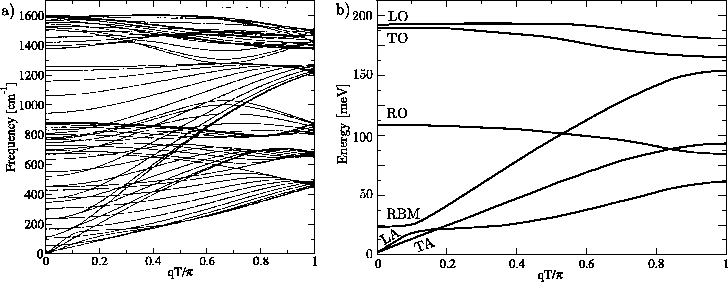M. Pourfath: Numerical Study of Quantum Transport in Carbon Nanotube-Based Transistors